# Data tables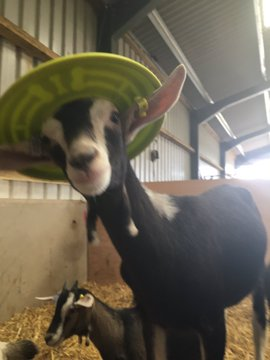Holly Vickery's friend

### What's a data table?

A data table is a bunch of rows and columns. For example, here's a data table about goats.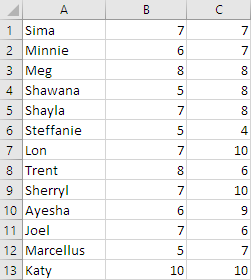Each row is about one thing. The first row is data about a goat.The second row is data about a different goat.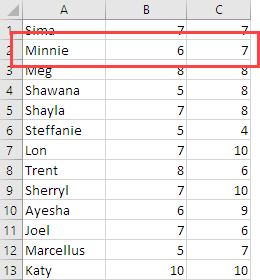Rows are also called records. Sometimes they're called cases. We'll stick with records, since that's a common term.

So, a data table is a list of records. Each record is about one thing.

Each column is usually called a field, or attribute. The first field is goat name.The second field is the goat's happiness level.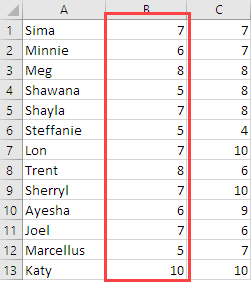The third field is coolness level.

So, a record is data about one goat. A field is an attribute that all goats have.

Each field has a data type. Usually, every value in a field is the same data type. Here, every goat name is a string. The others are numbers.

### Where you find data tables

Data tables are everywhere in MIS. They're in Excel, as you've just seen. They're in (comma-separated values) CSV files:

• "Sima",5,5
• "Minnie",5,7
• "Meg",6,9
• "Shawana",8,7
• "Shayla",8,10
• "Steffanie",7,6
• "Lon",8,9
• "Trent",6,7
• "Sherryl",4,7
• "Ayesha",8,4
• "Joel",9,5
• "Marcellus",7,7
• "Katy",5,8

In the CSV file, each row is a record. Each item is a field.

Data tables are in databases, like Access, SQL Server, or MySQL. For example, here's the same data from a MySQL database:The data-table-records-fields thing is easy for people to work with, so they turn up a lot.

### Data table processing pattern

There's a general pattern for processing data tables:

• Grab the first row
• Do something with it
• Grab the next row
• Do something with it
• Keep going until you run out of rows

Here's a data table. It's different from the one above:Data about four different goats. Three fields for each goat:

• Name
• Weight
• Whether the goat likes Borderlands

So, the pattern is to start at the top, and process the first row.Then the next.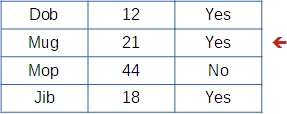Then the next.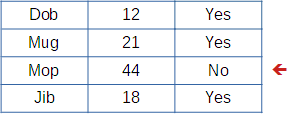Then the next.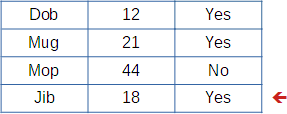Let's write in some record numbers, to make this easier to think about.It's useful to have the idea of a current record. When you're processing this record...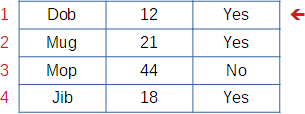... the current record is 1.

Imagine we made a variable called, say, `sCurrentRecordNumber`. It holds..., er, the current record number. It would start at one:When you're processing the next record...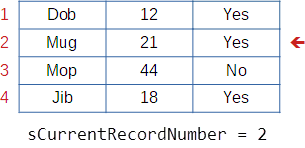Then...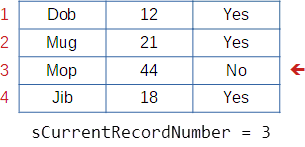Then...`sCurrentRecordNumber` points to the record being processed.

### Loops

A loop is code that's executed again and again, usually with something changing each time.

Here's the data again:Let's say that the second column is the goat's weight, in kilos. Suppose you've put each goat's weight into a variable called `sGoatWeight`. We'll look at the deets later.

We want to work out the total weight of all of the goats. Here's some code for that. You don't need to understand the deets, just get a general idea of what a loop is.

1. sTotal = 0
2. For sCurrentRecordNumber = 1 To 4
3.   sGoatWeight = [Goat weight for record number sCurrentRecordNumber]
4.   sTotal = sTotal + sGoatWeight
5. Next sCurrentRecordNumber
6. MsgBox "Total goat weight: " & sTotal

The loop is from lines 2 to 4. Line 3 is the body of the loop.

Line 3 is run again and again. The code never changes as the program runs. But what line 3 does changes, because `sCurrentRecordNumber` changes.

Here's what happens.

• Set `sCurrentRecordNumber` to 1, and run line 3.
• Set `sCurrentRecordNumber` to 2, and run line 3.
• Set `sCurrentRecordNumber` to 3, and run line 3.
• Set `sCcurrentRecordNumber` to 4, and run line 3.

In that list, let's take out "run line 3," and write in what line 3 is.

• Set `sCurrentRecordNumber` to 1. Run sTotal = sTotal + [Goat weight for record number sCurrentRecordNumber].
• Set `sCurrentRecordNumber` to 2. Run sTotal = sTotal + [Goat weight for record number sCurrentRecordNumber].
• Set `sCurrentRecordNumber` to 3. Run sTotal = sTotal + [Goat weight for record number sCurrentRecordNumber].
• Set `sCurrentRecordNumber` to 4. Run sTotal = sTotal + [Goat weight for record number sCurrentRecordNumber].

Now let's replace `sCurrentRecordNumber` with the value it has each time through the loop.

• Set `sCurrentRecordNumber` to 1. Run sTotal = sTotal + [Goat weight for record number 1].
• Set `sCurrentRecordNumber` to 2. Run sTotal = sTotal + [Goat weight for record number 2].
• Set `sCurrentRecordNumber` to 3. Run sTotal = sTotal + [Goat weight for record number 3].
• Set `sCurrentRecordNumber` to 4. Run sTotal = sTotal + [Goat weight for record number 4].

Here's the table again:Let's plug in the weight values from the table.

• Set `sCurrentRecordNumber` to 1. Run sTotal = sTotal + 12. sTotal is 0 + 12 -> 12.
• Set `sCurrentRecordNumber` to 2. Run sTotal = sTotal + 21. sTotal is 12 + 21 -> 33.
• Set `sCurrentRecordNumber` to 3. Run sTotal = sTotal + 44. sTotal is 33 + 44 -> 77.
• Set `sCurrentRecordNumber` to 4. Run sTotal = sTotal + 18. sTotal is 77 + 18 -> 95.

This is called the accumulator pattern. `sTotal` accumulates, as the loop is run. More later.

Pattern

Loop over a data table. For each record, add a field to a total, count, or whatevs.

To summarize, we run this line again and again:

• sTotal = sTotal + [Goat weight for record number sCurrentRecordNumber]

The value of `sCurrentRecordNumber` changes each time. It starts with the first record number (that's 1), then the next, then the next, and so on. By the end, the line...

• sTotal = sTotal + [Goat weight for record number sCurrentRecordNumber]

... has been run for every record.Ray

I get the general idea. But I don't know if I got it all.

That's OK. What's the general idea?Ray

There's a table of records. The loop has some code that's run for each record. First record, second record, until you get to the end. Is that right?

Yes, that's exactly right.

How does the code know which record it's working on, each time through the loop?Ray

Well, there's a variable, called `sCurrentRecordNumber`. It starts out at 1, then is 2, then 3, and so on.

The code grabs whatever record `sCurrentRecordNumber` is pointing to. Record 1, record 2, and the rest.

Perfect! That `sCurrentRecordNumber` points to a record is the key idea.

That's all you need to know at the moment.

Loops can take a while to understand. Up until now, your code has run from the top of your program, to the bottom. Now, we have some code that spins around and around, churning through the data.

### Up next

Let's get working on Ranges.